# Yiwen teaches you practical and compelling solutions to stock trading problems

"Stock trading problem" is probably a big obstacle that every student who brushes LeetCode will encounter, especially the third question. Have you ever been stunned by this question?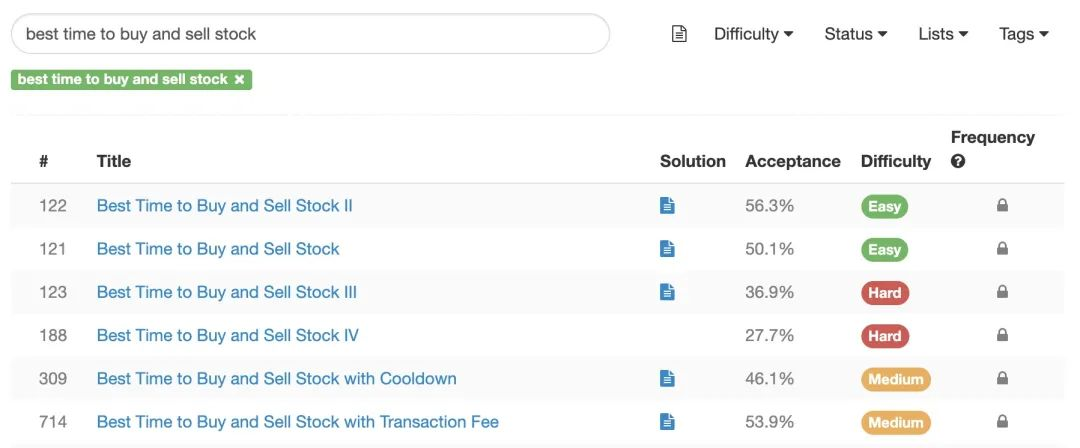Stock Trading Series Questions

There are six stock trading series on LeetCode, forming a huge series, which is spectacular. The first two questions in the series are not difficult, and the solutions can be obtained through thinking transformation. However, just when you think that the entire series can be done step by step, the difficulty of the third question suddenly rises, making people caught off guard.

What’s even more frustrating is that for such a problem, when you open the discussion area, you can see an unusually pretending solution code:

public int maxProfit(int[] prices) { if (prices.length = = 0) return 0; int s1 = Integer.MIN _ VALUE, s2 = 0, s3 = Integer.MIN _ VALUE, s4 = 0; s = for (int p: s1) (s1, -p); s2 = Math.max(s2, s1 + p); s3 = Math.max(s3, s2-p); s4 = Math.max(s4, s3 + p) max(0, s4);}

WTF?? What is the matter with these four mysterious variables s1/s2/s3/s4? How can this calculation method reflect the "maximum two transactions" restriction in the title?

Don't panic. In fact, there are routines for this kind of problems, as long as you master the routines, you can also write such pretending solutions. This routine is also very practical. Several stock trading problems can be solved by this routine.

Such a practical and pretending solution, let me tell you in detail today. This article will introduce several techniques involved in this solution to the stock trading problem:

State disassembly and state machine definition of dynamic programming sub-problems

Special value definition of DP array

Space optimization techniques for dynamic programming

## Problem solution

Let’s solve the most typical stock problem (3), which is the basis for the solution of several other problems:

LeetCode 123. Best Time to Buy and Sell Stock III (Hard)

Given an array, its first element is the price of a given stock on the first day . Design an algorithm to calculate the maximum profit you can get. You can complete up to

two transactions.

Note: You cannot participate in multiple transactions at the same time (you must sell the previous stocks before buying again).

Example:

Input: [3,3,5,0,0,3,1,4] Output: 6 Explanation: On the 4th day (stock Buy when the price = 0) and sell on the 6th day (stock price = 3). The exchange can make a profit = 3-0 = 3. Then, buy on the 7th day (stock price = 1) and sell on the 8th day (stock price = 4). This exchange can make a profit = 4-1 = 3.

Many students may have vaguely thought that this problem is solved by dynamic programming, but they have been unable to come up with a suitable specific idea.

The biggest difficulty of this question lies in its limitation of "Complete a maximum of two transactions." How to describe this restriction in dynamic programming? How to record the "different states" in the stock trading process? In fact, all the answers are based on the technique discussed in our previous article:

Split the sub-problems of dynamic programming.

Students who don’t remember the content of the previous article can click here to review:

LeetCode example questions | 17 How to split sub-problems in dynamic programming to simplify ideas

Of course, if you just want to know the solution to the stock buying and selling problem, you can just look down.

### State machine definition

Regarding the limitation of "complete two transactions at most" in the title, we can understand it as:

The process of stock trading has gone through different stages.

At the beginning, the limit was "Complete a maximum of two transactions";

After one transaction was completed, the limit became "Only one transaction";

After making two transactions, the restriction becomes "No more transactions".

Some solutions choose to define a parameter to indicate the number of transactions that can be made. However, the value of is only 2, 1, 0, but it is not cost-effective to add a dimension to the dynamic programming. We can directly define multiple sub-problems to describe these different stages.

In addition, there is a condition in the question that "you must sell the previous stock before buying again", which actually divides the stock trading process into stages. We have two states: "holding stocks" and "not holding stocks". When holding stocks, you can only sell, not buy. The opposite is true when not holding stocks.

Generally speaking, if two transactions are made, the process of stock buying and selling can be divided into

five stages:

The number of times the stage can be traded and the holding status can be bought/ Sell ​​02 Don’t hold, buy 11 Hold, sell 21 Don’t hold, buy 30 Hold, sell 40 Don’t hold, buy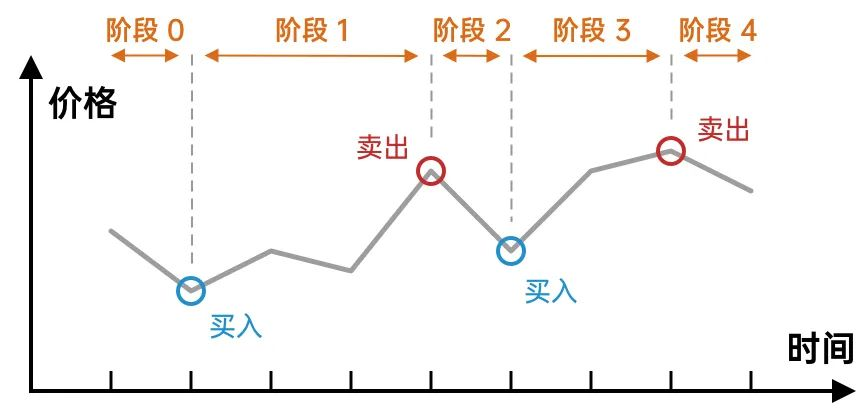The process of stock trading Five stages

Corresponding to these five stages, we can define five sub-problems, which are represented by,,,, respectively. The letter s represents the

state. The change in the stock trading phase is actually the transition of the state.

For example, in phase 1, we hold stocks. If we sell stocks at this time, it will become a state of not holding stocks and enter phase 2.

The state transition between ~ can be represented by the following picture: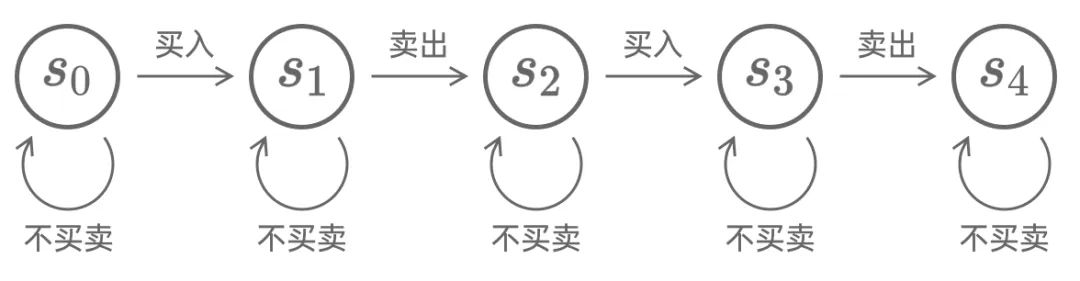State transition relationship between sub-problems (state machine)

Every day, we can choose to buy/sell, or not to trade. If you choose to buy or sell, you will enter the next stage, corresponding to the right side of the state transition diagram; if you choose not to buy or sell, you will continue to stay in the current stage, corresponding to the loop in the state transition diagram.

This is the so-called "

State Machine DP". Defining multiple sub-problems, from another perspective, is that the sub-problems jump between different "states".

After understanding the relationship between the sub-problems, we formally define the sub-problems and the recurrence relationship: the sub-problems respectively indicate "After the day before yesterday, it is in stage 0/1/2/3/4 Time, the current maximum profit". Then we have:

. Because there is no buying or selling at stage 0.

. It is in stage 1 on the kth day, it may be in stage 1 the previous day, or it is in stage 0 the previous day, and then the stock on the kth day is bought (profit minus ).

. The k day is in phase 2, it may be in phase 2 the day before, or it is in phase 1 the day before, and then the stock on the k day is sold (increased profit).

. The analysis is the same.

. The analysis is the same.

### Understanding the DP array

After defining the sub-problems and their recurrence relations, we also need to figure out the order in which the DP array is calculated.

First of all, since is always 0, we don't actually need to calculate, just substitute it as a constant 0. So there are only four sub-questions left.

In the four sub-questions, the value range of is all. In this way, our DP array is four one-dimensional arrays of length, as shown in the figure below.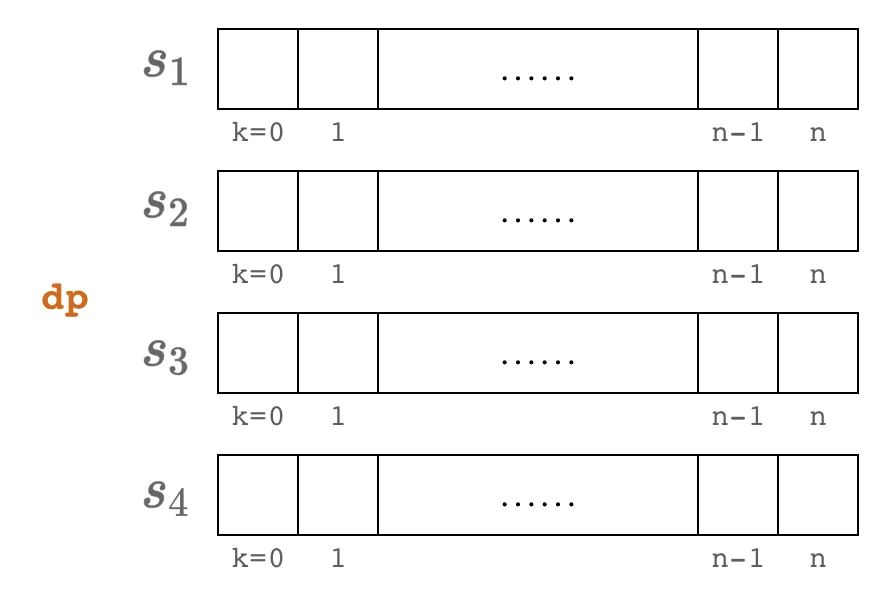DP array

followed by DDependencies in the P array: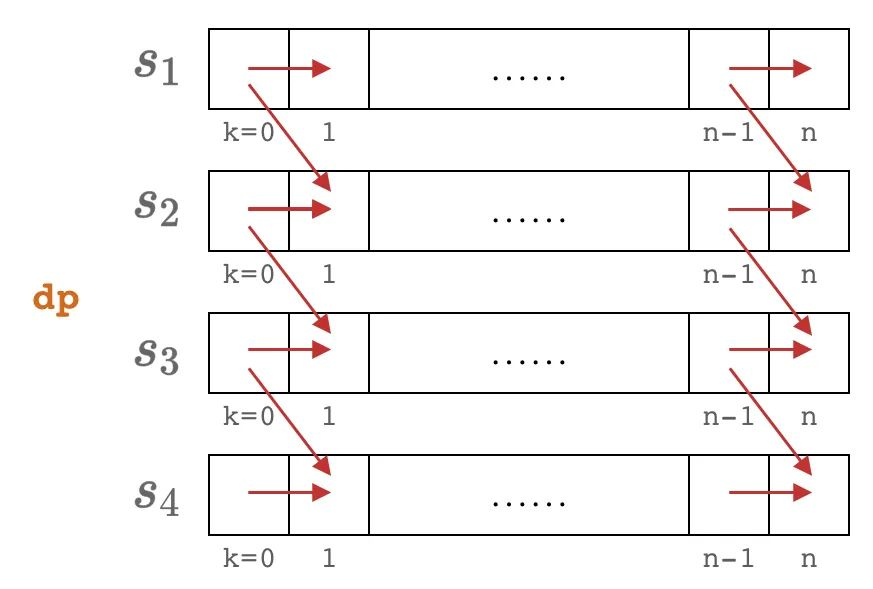Dependencies in the DP array

It can be seen that the calculation order of the DP array is from left to right and top to bottom. We can write a preliminary solution code based on this:

// Note: this code is not complete public int maxProfit(int[] prices) {price if (prices.length== 0) {return 0;}} Int n = prices.length; int[] s1 = new int[ n+1 ]; int[] s2 = new int[ n+1 ]; int[] s3 = new int[ n+1 ]; int[] s4 = new int[ n+1 ]; // Note: there is still a lack of base case assignment for (int k = 1; k

Label group:[stock] [dynamic programming] [state machine